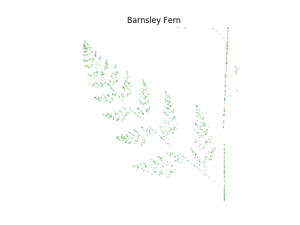# Barnsley Fern in Python

Feb, 18, 2017

The Barnsley Fern is a beautiful fractal that can easily be generated in Python.If we zoom in on one branch, we see that the pattern is repeated:The python code follows.

```from __future__ import division, print_function
import numpy as np
import matplotlib.pyplot as plt

# Main body of program
points = 10000  # The number of points to use.

X = []  # A list of x-coordinates
Y = []  # A list of y-coordinates

# Set the starting point
point = [0.5, 0.0]
X.append(point)
Y.append(point)

def new_point(p):
"""
This function takes in a point (x, y) and generates a new point according
to the given equations.
"""
r = np.random.uniform(0, 1)
if r < 0.02:
p = [0.5, 0.27*p]
elif 0.02 <= r <= 0.17:
p = [-0.139*p + 0.263*p + 0.57, 0.246*p + 0.224*p - 0.036]
elif 0.17 < r <= 0.3:
p = [0.17*p - 0.215*p + 0.408, 0.222*p + 0.176*p + 0.0893]
elif 0.3 < r < 1.0:
p = [0.781*p + 0.034*p + 0.1075, -0.032*p + 0.739*p + 0.27]

return p

# Generate a large number of points
for i in range(points):
point = new_point(point)
X.append(point)
Y.append(point)

# Plot the results
plt.scatter(X, Y, c='g', s=.05)
plt.axis('Off')
plt.axes().set_aspect('equal')
plt.title("Barnsley Fern")
plt.savefig("barnsley_fern.png")
plt.show()

```
Posted in Python

This site uses Akismet to reduce spam. Learn how your comment data is processed.

Follow